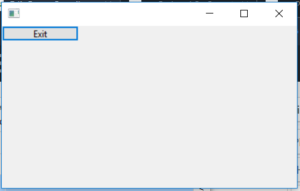GFG App
Open AppBrowser
Continue

# wxPython | Exit() function in wxPython

In this article we are going to learn about wx.Exit() which is a inbuilt parent function present in wxPython.Exit() function exits application after calling wx.App.OnExit .

Should only be used in an emergency: normally the top-level frame should be deleted (after deleting all other frames) to terminate the application. See wx.CloseEvent and wx.App.

Syntax:
wx.Exit()

Parameters:
No parameters are required by Exit() function

Coding Example:

 `import` `wx ` ` `  ` `  `class` `Example(wx.Frame): ` ` `  `    ``def` `__init__(``self``, ``*``args, ``*``*``kwargs): ` `        ``super``(Example, ``self``).__init__(``*``args, ``*``*``kwargs) ` ` `  `        ``self``.InitUI() ` ` `  `    ``def` `InitUI(``self``): ` ` `  `        ``self``.locale ``=` `wx.Locale(wx.LANGUAGE_ENGLISH) ` `        ``self``.panel ``=` `wx.Panel(``self``, pos ``=``(``100``, ``100``), size ``=``(``100``, ``100``)) ` `        ``self``.btn ``=` `wx.Button(``self``.panel, ``id` `=` `2``, label ``=``"Exit"``, pos ``=` `wx.DefaultPosition, size ``=``(``100``, ``20``)) ` ` `  `        ``self``.Bind(wx.EVT_BUTTON, ``self``.onclick, ``self``.btn) ` ` `  `    ``def` `onclick(``self``, e): ` `        ``# EXITS APPLICATION ON CLICKING EXIT BUTTON ` `        ``wx.Exit() ` ` `  `def` `main(): ` `    ``app ``=` `wx.App() ` `    ``ex ``=` `Example(``None``) ` `    ``ex.Show() ` `    ``app.MainLoop() ` ` `  ` `  `if` `__name__ ``=``=` `'__main__'``: ` `    ``main() `

Output Window:My Personal Notes arrow_drop_up